## The PREP_MESH application

### Presentation

This application is controlled by the application menu on top of the screen (figure 1.26) and the selection menu on bottom of the screen (figure 1.19). It is used to define:

• the discretization of the contour lines,
• the subdomains to be meshed,
• the references of the lines and their extremities, with the objective of taking boundary conditions into account (cf. the finite elements method),
• the type of mesh generator, either structured (deformed quare) or non structured (Voronoi), for each subdomain.

Furthermore, at this point, we have the option of interfacing with the APNOPO  mesh generator by generating a data file for the APNOXX preprocessor, see secapnopo.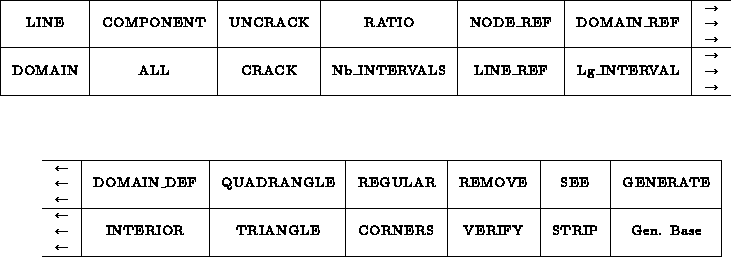Figure: The PREP_MESH application menu

### Utilization

When we enter this application, the program automatically calculates all the interior and exterior connex components of the design, for example figure 1.27. The interior connex components are oriented counterclockwise and the exterior connex components are oriented clockwise.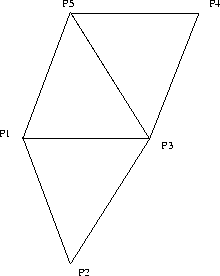Figure 1.27: Example of components

We thus obtain automatically the following connex components:

```   P5,P3,P4       >0
P1,P3,P5       >0
P1,P2,P3       >0
P1,P5,P4,P3,P2 <0
```

• We will call <element>  an ARC, a SEGMENT or a SPLINE.
• We will call <component>  a component selected through one of its element.
• We will call <domain>  a domain selected through one of its elements
(component or interior element).
• We will call <line>  = LINE element1 element2 the set of all elements going from the first element element1 to the second element element2 in the orientation of the component to which both elements belong.

#### Modification of the state of the system

The state of the system  is a set of variables used by the application. The global variables of the application are:
• %RATIO,  used to define the ratio of the discretization of a curve (line, segment, arc or spline)
• %NBINTR,  used to define the number of intervals of discretization of a curve
• %LGINTR,  used to define the length of the of intervals of discretization of a curve
• %NUREF,  used to define a reference number for a point, a curve or a subdomain.
These variables can be modified at almost any moment.

We will call <state modif>  a modification of the state of the system, which is to say a modification of the value assigned to one of the variables.

```<state modif>  = *( <ratio> | <nb interval> | <lg interval> | <ref number> )
```

• Modification of %RATIO: <ratio>  = RATIO  ( <VALUE>  | IDEM)
• Modification of %NBINTR: <nb interval>  = NBINTR  (VALUE | IDEM)
• Modification of %LGINTR: <lg interval>  = LGINTR  (VALUE | IDEM)
• Modification of %NUREF: <ref number>  = NUREF  (VALUE | IDEM)
IDEM keeps the previous value of the state variable.

#### Definition of intermediate points

```    Nb_INTERVAL * <VALUE>
* (   LINE       *(( <VALUE> | ")<element1> <element2>)
| COMPONENT  *(( <VALUE> | ")<element3>)
| ALL
|              ( <VALUE> | ")<element4>  )
```
This command is used to generate intermediate points on
• the elements of a line defined by element1 element2
• the elements of a component selected by element3
• all the elements of the D.B.
• only one element element4
so as to obtain %NB_INTERVAL  intervals. If VALUE is entered, %NB_INTERVAL takes this value and it is displayed on the bottom line of the screen.

```   Lg_INTERVAL  *<VALUE>
* (    LINE        *(( <VALUE> | ")<element1> <element2>)
| COMPONENT    *(( <VALUE> | ")<element3>)
| ALL
|                ( <VALUE> | ")<element4>  )
```
This command is used to generate intermediate points on
• the elements of a line defined by element1 element2
• the elements of a component selected by element3
• all the elements of the D.B.
• only one element element4
in such a way that the distance between two consecutive points is close to %Lg_INTERVAL . If VALUE is entered,
 %Lg_INTERVAL takes this value and it is displayed on the bottom line of the screen.

#### Definition of the ratio

```    RATIO  * <VALUE>
* (   LINE      *((  <VALUE> | ")<element1> <element2>)
| COMPONENT *((  <VALUE> | ")<element3>)
| ALL
|              ( <VALUE> | ")<element4>  )
```

This command is used to assign %RATIO  as ratio to

• the elements of a line defined by element1 element2
• the elements of a component selected by element3
• all the elements of the D.B.
• only one element element4.
If VALUE is entered, %RATIO takes this value and it is displayed on the bottom line of the screen.

#### Definition of the reference number of extremities

```    NODE_REF * <VALUE>
* (   LINE      *(( <VALUE>  | ")<element1> <element2>)
| COMPONENT *(( <VALUE>  | ")<element3>)
| DOMAIN    *(( <VALUE>  | ")<element4>)
| ALL
|              ( <VALUE>  | ")<element5>  )
```
This command is used to assign %NUREF as reference number to

• the extremities of the elements of a line defined by element1 element2
• the extremities of the elements of a component selected by element3
• the extremities of the elements of a domain selected by element4
• all extremities of the elements of the D.B.
• the extremities of only one element element5

If VALUE is entered, %NUREF takes this value and it is displayed on the bottom line of the screen.

If the selected elements are cracks, VALUE will be assigned to the extremity and on the side where the element, the LINE or the COMPONENT was clicked, and by continuity to the extremities of the adjacent elements. In effect, a crack element possesses a line reference number and two extremity reference numbers on its left and/or on its right. These numbers can be identical or different. If they are different, then the element is necessarily a crack.

#### Definition of the line reference number

```    LINE_REF * <VALUE>
* (   LINE      *(( <VALUE>  | ")<element1> <element2>)
| COMPONENT *(( <VALUE>  | ")<element3>)
| DOMAIN    *(( <VALUE>  | ")<element4>)
| ALL
|              ( <VALUE>  | ")<element5>)
```
This command is used to assign %NUREF  as reference number to

• the elements of a line defined by element1 element2
• the elements of a component selected by element3
• the elements of a domain selected by element4
• all the elements of the D.B.
• only one element element5.

If VALUE is entered, %NUREF takes this value and it is displayed on the bottom line of the screen.
If the selected elements are cracks, %NUREF will be assigned to the side on which the element, the LINE or the COMPONENT was clicked.

#### Cracking lines

```    CRACK   * (   LINE      *(<element1><element2>)
| COMPONENT * <element3>
| ALL
|              <element4>  )
```
This command is used to crack a line in a mesh (the two side of a line a different in the mesh)
• the elements of a LINE defined by element1 element2
• the elements of a COMPONENT selected by element3
• all the elements of the D.B.
• only one element element4.

#### Uncracking lines

```    UNCRACK * (   LINE      *(<element1><element2>)
| COMPONENT * <element3>
| ALL
|              <element4>  )
```
This command is used to uncrack
• the elements of a LINE  defined by element1 element2
• the elements of a COMPONENT  selected by element3
• all  the elements of the D.B.
• only one element element4.
This the inverse of the cracking operation. The number of references is brought down to 1 by only retaining the left reference number of the crack.

#### Definition of a subdomain reference number

```    DOMAIN_REF *( <VALUE>  | <domain> | ALL )
```
This command is used to assign VALUE as subdomain reference number to the selected domain DOMAIN or to all defined domains. If DOMAIN does not exist, it is created.

#### Defining a domain

```    DEF_DOMAI <element1>
* (<element2> | INTERIOR *(<point> | <element3> | <line>) )
```
• If element1 is not already included in a domain, then we create a domain with number %NUREF that has element1 as exterior component and whose interior components are selected by the following elements element2 if any or rather, the interior elements are defined by INTERIOR followed by point or the intermediate points of element3 or the intermediate points of the elements of the line.
• If element1 is already included in a domain then the component selected by element2 is added to the components of the domain as an interior component.

#### Adding interior elements to a domain

```    INTERIOR *( <element1> *( <point> | <element2> | <line>) )
```

The INTERIOR command is used to add interior elements to a domain selected by the element element1. The interior elements that can be added to a domain are point or the intermediate points of element2 or the intermediate points of the elements of line.

#### Verification of the correct definition of a domain

```    VERIFY  *( ALL | <element>)
```
• This command is used to verify if the domain selected by element has been defined correctly.
• It is used to verify if all domains are defined correctly, for example to check that no interior components of negative surface are present, etc.

#### Removing elements from a domain

```    REMOVE  *(   INTERIOR *( <point> | <element1>)
| DOMAIN *<element2>
| ALL
| <element3> )
```
This is used to remove
• the element element1 or an interior point in a domain selected by this element1 or this point
• a domain selected by element2
• all domains
• the component selected by element3 of a domain. It is not possible to apply this command if the component is exterior because the first hole component would then become exterior, cf. internal structure of the data.

#### Meshing mode of a domain

```    TRIANGLE    *<domain>
```
Requests that the domain be divided into triangles  by using a Voronoi algorithm 

```    QUADRANGLE  *(<domain>
(  <line>
| <element>
| (  EXTREMITY<element1> EXTREMITY<element2>
EXTREMITY<element3> EXTREMITY<element4> )
| " ) )
```
Requires that the domain be homeomorphic to a square (deformed quadrangle ). The command requests that the quadrangular subdomain should contain a structured, NxM finite difference type mesh like in figure 1.28. It will be divided into quadrangles, unless we change the partition mode with (1.5.4) if you want to use the GENERATE item 1.5.4. There are two ways of describing the quadrangular domain, by the four edges or the four vertices:
• the first edge of the domain is defined by line line or by element element,
• we enumerate the four vertices of the domain by giving the four extremities of the elements that define them. The vertices must be enumerated counterclockwise .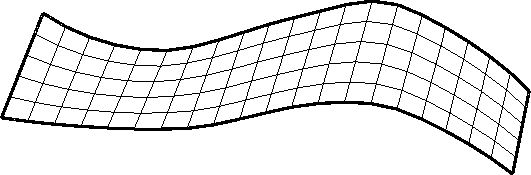Figure 1.28: Example of QUANDRANGLE mesh: nb. points: on right and left edges is 6=N, and on lower and upper edge is 21=M

```    STRIP  *(<domain>
(  <line>
| <element>
| (  EXTREMITY<element1> EXTREMITY<element2>
EXTREMITY<element3> EXTREMITY<element4> )
| " ) )
```
Requires that the domain be homeomorphic to a square (deformed quadrangle) with an equal number of points N over a couple of opposite edges, like in figure 1.29 ( the case quadrangle requires the equality of numbre of points over the two couple of opposites edges , the `` quadragle mesh'' generator is always used in this case). (N-2) internal lines are generated, joining correspondind points of opposite edges and points are distributed on these lines using interpolation procedures. These (N-2) internal lines definie (N-1) strips which are divided into triangle using a special option of the mesh generator (no extra internal points are generated).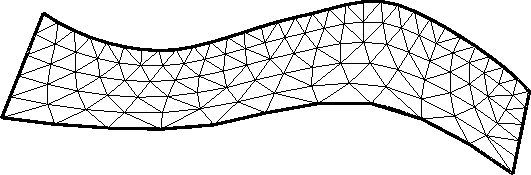Figure 1.29: Example of STRIP mesh, nb. of node: on right and left edges is 6 = N , on lower edge is 11, and on upper edge is 31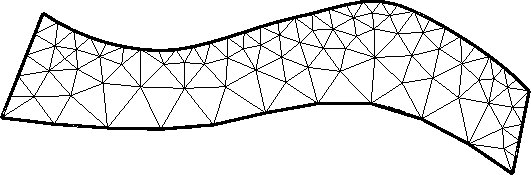Figure 1.30: Example of Delaunay-Voronoi mesh with the same input than in the previous figure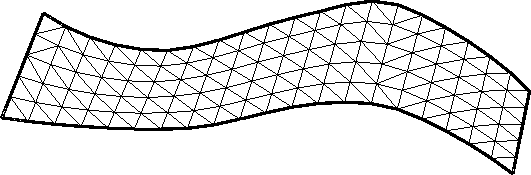Figure 1.31: Example of STRIP mesh (Quadrangle mesh), nb. of nodes: on right and left edges is 6 = N , and on lower and upper edge is 21

There are two ways of describing the strip domain, by the four edges or the four vertices:

• the first edge of the domain is defined by line line or by element element,
• we enumerate the four vertices of the domain by giving the four extremities of the elements that define them. The vertices must be enumerated counterclockwise .

Remark: This kind of mesh generator is may be useful of the boundary layer region.

#### Partition mode for a quadrangular domain

```    REGULAR  *(<domain> <VALUE> )
```
Requests that the partition of quadrangles into triangles in the domain be, see the MODULEF APNOPO booklet:
• regular on the right (value=2)
• regular on the left (value=1)
• non regular (value=0)
depending on the value.
```    CORNERS  *(<domain> <VALUE> )
```
Requests that the partition of quadrangles into triangles in the domain be effected taking or not the corners into account, depending on the value ( Yes = 0 / No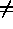0).

#### Visualization of domains or components

```    SEE    DOMAIN *(<domain> | <component> | ALL )
```
This command is used to visualize using thick lines the domain or the componant selected by one of its elements. This operation is useful for debugging a mesh (problems with intersection, round off error).

#### Generating an APNOXX input file

```   GENERATE
```
This command is used to generate an input file for APNOXX (Modulef).
• The input file will be assigned the name XXX by the user followed by the suffix .DATA.
• The input file will request APNOXX to generate a NOPO titled XXX.NOPO.
The program takes into account only those domains which have been previously defined.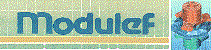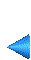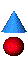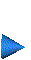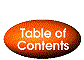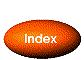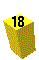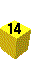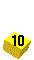Next: The EDIT_MESH application Up: The EMC˛ preprocessor Prev: 1.5.3 The construction application Index Contents